# 早下班系列：比python更称手的兵器-续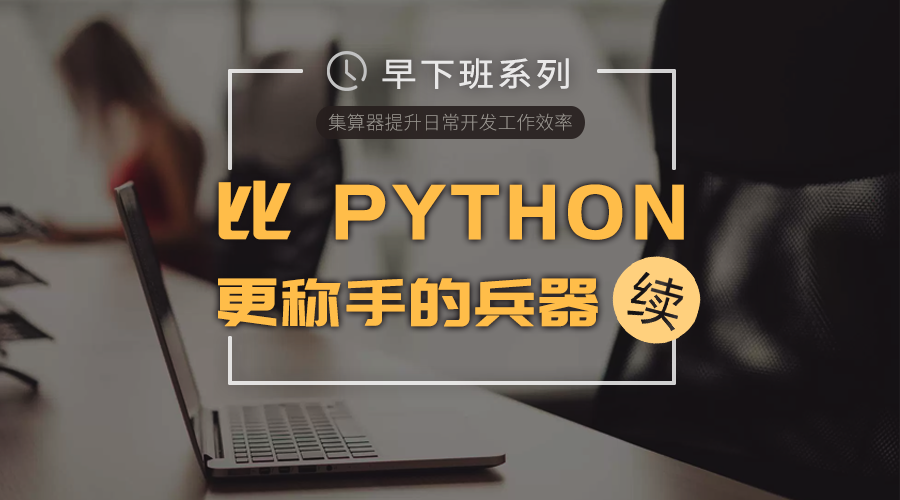import pandas as pd

data.PRICE = data.PRICE.str[1:].str.replace(',','').astype('int64')

out = data.groupby(['STYLE','BEDROOMS']).mean()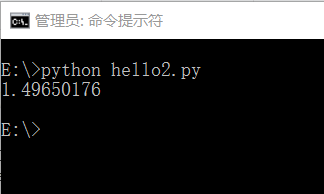## 一、玩一点真正的大数据计算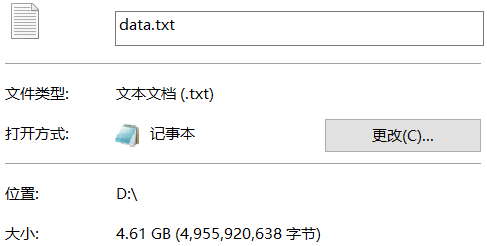A 1 =file("D:/data.txt").cursor@t(#1,#2:decimal,#3:int,#4:decimal,#5;," ") 2 =A1.run(decimal(replace(replace(#5,"\$",""),",","")):PRICE) 3 =A2.groups(STYLE,BEDROOMS;avg(SQFEET):SQFEET,avg(BATHS):BATHS,avg(PRICE):PRICE)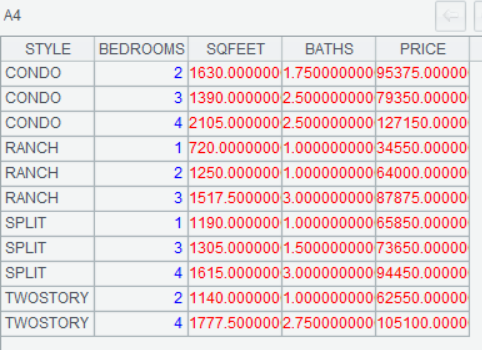import pandas as pd

data.PRICE = data.PRICE.str[1:].str.replace(',','').astype('int64')

print(data.groupby(['STYLE','BEDROOMS']).mean())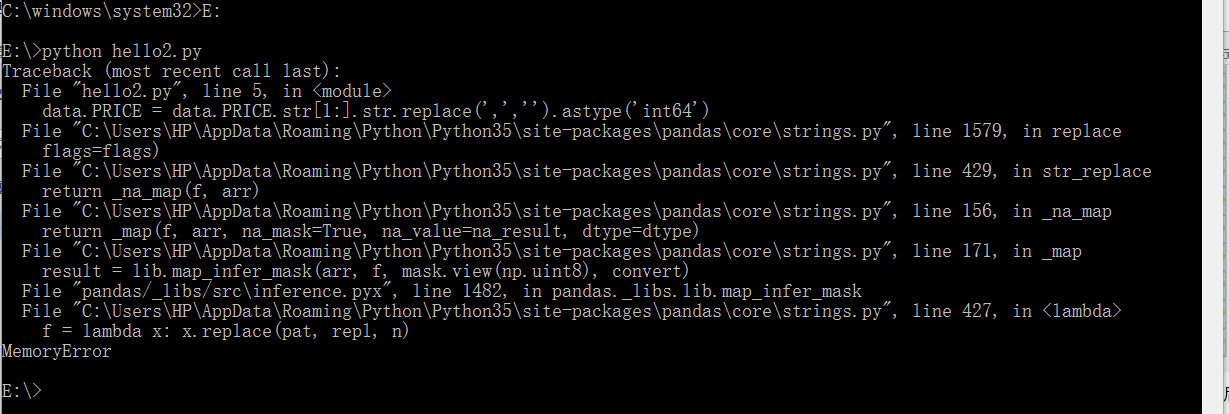## 二、读个其他类型的文件试试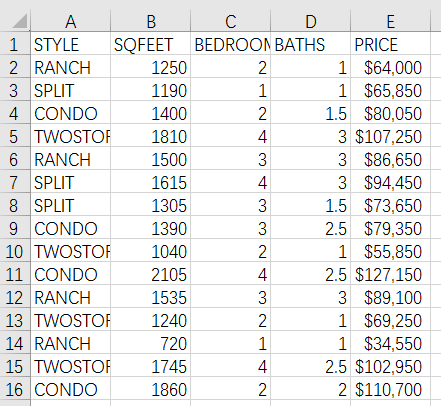A 1 =file("D:/data.xlsx").importxls@tc() 2 =A1.groups(STYLE,BEDROOMS;avg(SQFEET):SQFEET,avg(BATHS):BATHS,avg(PRICE):PRICE)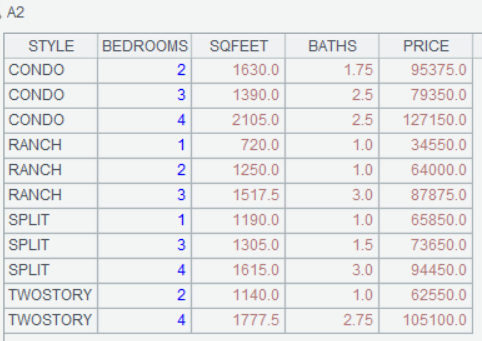import pandas as pd

print(data.groupby(['STYLE','BEDROOMS']).mean())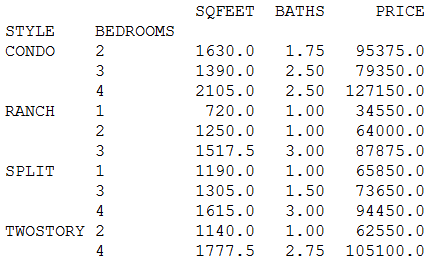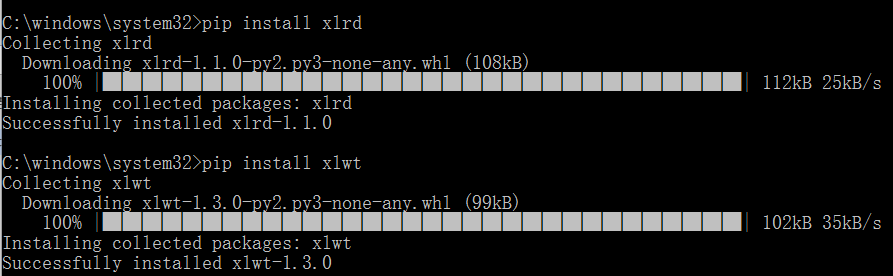## 三、算一点稍微复杂的东西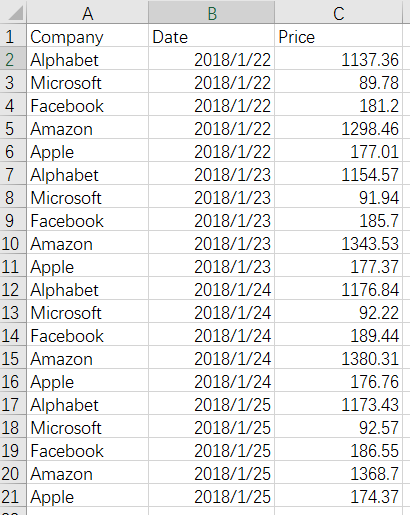A 1 =file("E:/Stock.xlsx").importxls@t().sort(Date).group(Company) 2 =A1.((a=0,~.max(a=if(Price>Price[-1],a+1,0)))) 3 =string(A2.count(~>=4)/A2.count(~>=3),"0.00%")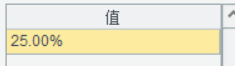import pandas as pd

def iterate(col):

prev = 0;

res = 0;

val = 0;

for curr in col:

if curr - prev > 0:

res += 1;

else:

res = 0;

prev = curr;

if val < res:

val = res;

return val;